# Coordinate axes

Determine the area of the triangle given by line -7x+7y+63=0 and coordinate axes x and y.

S =  40.5

### Step-by-step explanation: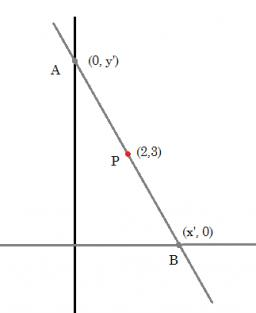Did you find an error or inaccuracy? Feel free to write us. Thank you!Tips to related online calculators
Line slope calculator is helpful for basic calculations in analytic geometry. The coordinates of two points in the plane calculate slope, normal and parametric line equation(s), slope, directional angle, direction vector, the length of the segment, intersections of the coordinate axes, etc.
Do you want to convert length units?

#### You need to know the following knowledge to solve this word math problem:

We encourage you to watch this tutorial video on this math problem:

## Related math problems and questions:

• XY triangleDetermine the area of a triangle given by line 7x+8y-69=0 and coordinate axes x and y.
• CircleCircle is given by centre on S[-7; 10] and maximum chord 13 long. How many intersect points have circle with the coordinate axes?
• Vertices of a right triangleShow that the points D(2,1), E(4,0), F(5,7) are vertices of a right triangle.
• Right triangle from axesA line segment has its ends on the coordinate axes and forms with them a triangle of area equal to 36 square units. The segment passes through the point ( 5,2). What is the slope of the line segment?
• Three pointsThree points A (-3;-5) B (9;-10) and C (2;k) . AB=AC What is value of k?
• ProveProve that k1 and k2 are the equations of two circles. Find the equation of the line that passes through the centers of these circles. k1: x2+y2+2x+4y+1=0 k2: x2+y2-8x+6y+9=0
• EllipseEllipse is expressed by equation 9x2 + 25y2 - 54x - 100y - 44 = 0. Find the length of primary and secondary axes, eccentricity, and coordinates of the center of the ellipse.
• Calculate 6Calculate the distance of a point A[0, 2] from a line passing through points B[9, 5] and C[1, -1].
• Polygon - area coordinatesFind the perimeter and the area of the polygon with the given vertices. T (2,7), U (2,9), V (5,9), W (5,7)
• On a lineOn a line p : 3 x - 4 y - 3 = 0, determine the point C equidistant from points A[4, 4] and B[7, 1].
• SquarePoints A[9,9] and B[-4,1] are adjacent vertices of the square ABCD. Calculate the area of the square ABCD.
• Right triangle - leg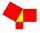Calculate to the nearest tenth cm leg length in the right-angled triangle with hypotenuse length 9 cm and 7 cm long leg.
• Lie/do not lie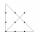The function is given by the rule f(x) = 8x+16. Determine whether point D[-1; 8] lies on this function. Solve graphically or numerically and give reasons for the your answer.
• Find the 5Find the equation of the circle with center at (1,20), which touches the line 8x+5y-19=0
• The angle of lines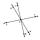Calculate the angle of two lines y=x-21 and y=-2x+14
• 3d vector componentThe vector u = (3.9, u3) and the length of the vector u is 12. What is is u3?
• Center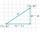Calculate the coordinates of the center of gravity T [x, y] of triangle ABC; A[11,4] B[13,-7] C[-17,-18].